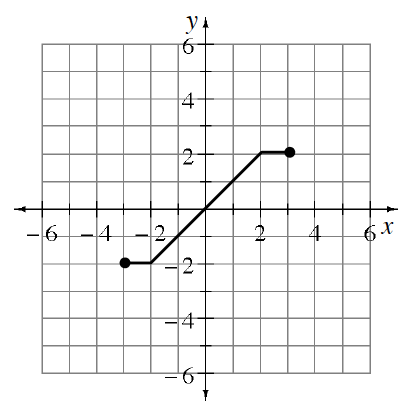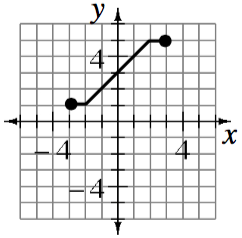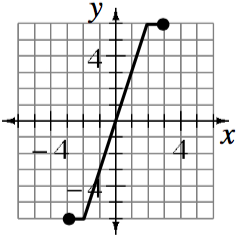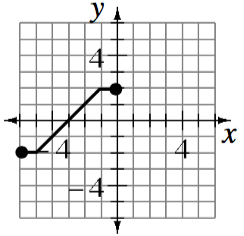### Home > INT2 > Chapter 9 > Lesson 9.3.2 > Problem9-98

9-98.Copy the graph of $y = f(x)$ at right onto graph paper, and then graph the following transformations.

1. $y = f(x) + 3$

2. $y = 3 · f(x)$

3. $y = f(x + 3)$

$y = f ( x ) + 3$ Piecewise graph, 3 sections, Connected as follows: starts at closed point (negative 3, comma 1), goes horizontal to the point (negative 2, comma 1), increases to the point (2, comma 5), & horizontal to the closed ending point, (3, comma 5).

$y = 3 \cdot f ( x )$ Piecewise graph, 3 sections, Connected as follows: starts at closed point (negative 3, comma negative 6), goes horizontal to the point (negative 2, comma negative 6), increases to the point (2, comma 6), & horizontal to the closed ending point, (3, comma 6).

$y = f ( x + 3 )$ Piecewise graph, 3 sections, Connected as follows: starts at closed point (negative 6, comma negative 2), goes horizontal to the point (negative 5, comma negative 2), increases to the point (negative 1, comma 2), & horizontal to the closed ending point, (0, comma 2).